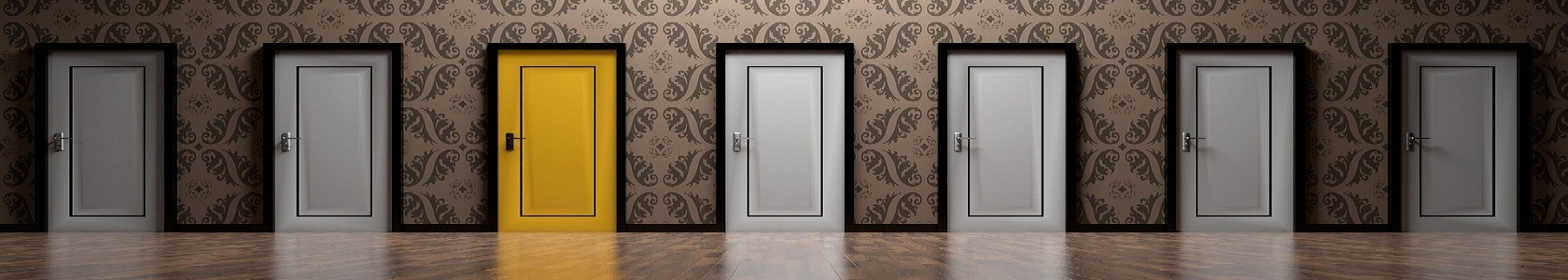# Nursing quiz

##### Nursing Research Quiz - 291 2 3 4 5 6 7 8 9 10 11 12 13 14 15 16 17 18 19 20 21 22 23 24 25 26 27 28 29 30

#### Nursing Research Quiz - 29

##### STATISTICAL ANALYSIS

1. Which of the following is NOT an assumption made by parametric tests?

A. Sample scores a have been drawn from a normally distributed population

B. The samples are assumed to have the same variance

C. DV is measured on an interval scale

D. DV is measured on an ordianal or nominal scale

2. Methods of checking assumption of normal distribution are:

A. Plotting data in the form of a histogram

B. Calculating skewness of the distribution (z-score + 1.96)

C. Calculating kurtosis of the distribution (z-score + 1.96)

D. Applying Kolmogorov-Smirnov test

E. All of the above

3. Which of the following statements about Box Plot are true?

In a Box Plot for variability,

A. the box represents the difference between the 25th and 75th percentiles.

B.  the larger the box, the greater the spread of the data.

C. the outliers are cases  with the values between 1.5 and 3 box-lengths from  the 75th percentile or 25th percentile.

D. the extreme values are cases with the values more than 3 box-lengths from the 75th percentile or 25th percentile.

4. Which of the following statements about normal distribution are true?

A. A value of zero for the skewness indicates a symmetric distribution.

B. The normal distribution has kurtosis of zero.

C. Shapiro-Wilk statistic for normality is calculated when the weighted sample size lies between 3 and 50.

D. Normal distribution is explained on the basis of mean and S.D

5. What is the appropriate sytatistical test for the following research question?

"Is there a difference in the confidence levels (measured on interval scale) of men and women who fall into one of three different age groups?"

A. One-way ANOVA

B. 2 by 3 Factorial ANOVA

C. Chi-square test

D. Multiple regression

6. Sphericity assumption in repeated measures one-way ANOVA is tested using the (SPSS):

A. Mauchly's test

B. t-test

C. Greenhouse-Geissor

D. LSD

7. Circularity/sphericity assumption is applicable in:

A. t-test

B. With-in-group one-way ANOVA

C. Between-group one-way ANOVA

D. Regression

8. What is NOT true about t-test?

A. As degrees of freedom increases, critical value of t decreases

B. Critical value for t at 0.10 level of significance for a two-tailed hypothesis is same as the critical value required for 0.05 level of significance with a one-tailed hypothesis

C. Critical value for t decrease as the sample size increases

D. Critical value for t is independent of the sample size.

9. What is NOT true about Yate's Correction?

A. Applied when there is two categorical variable are compared.

B. Applied when there is 2X2 contingency table is used

C. Recommended when there is less than 5 numbers are there is contingency cells

D. It is used when normality assumption is not satisfied.

10. For calculation of degree of freedom for chi-square test, the formula applied is:

A. n-1

B. n-2

C. (c-1) x (r-1)

D. k (n-1)